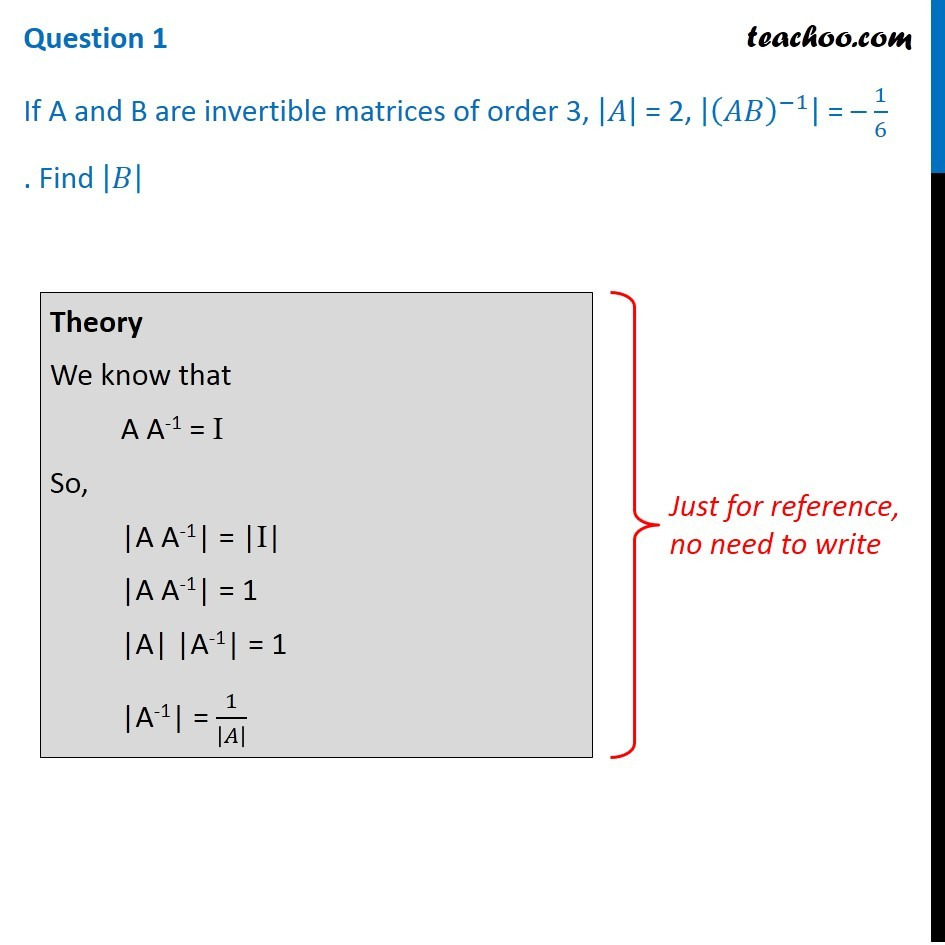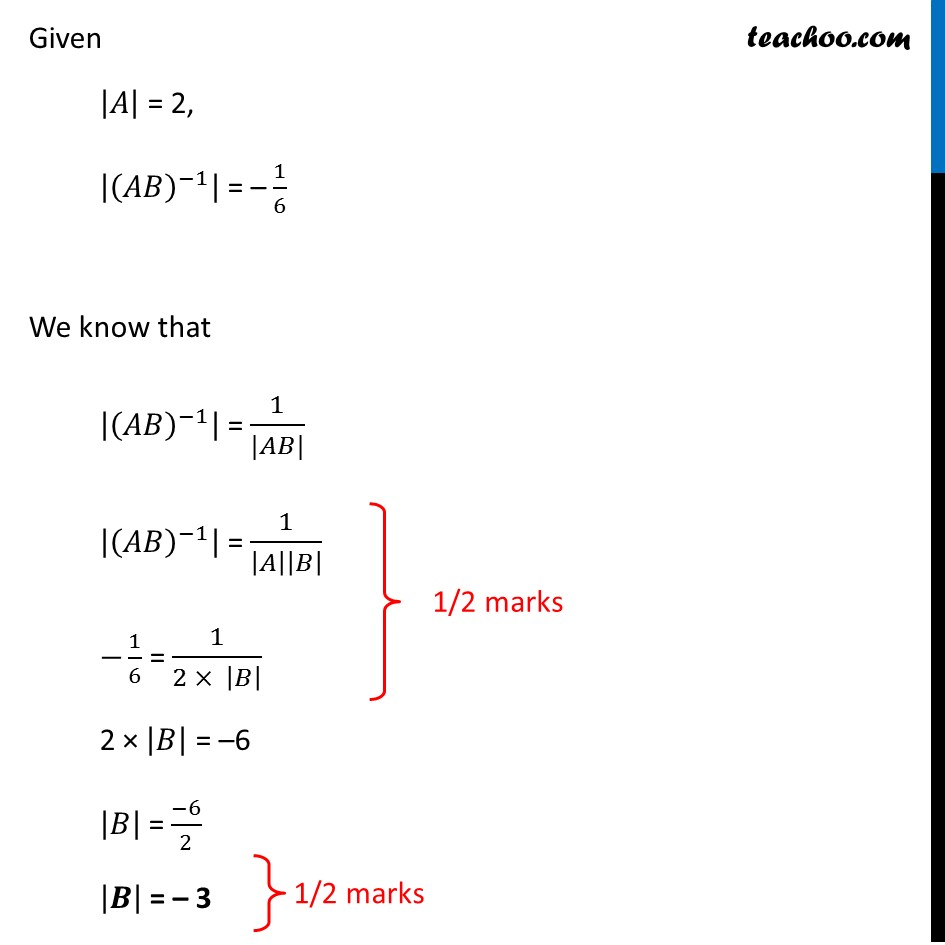CBSE Class 12 Sample Paper for 2019 Boards

Class 12
Solutions of Sample Papers and Past Year Papers - for Class 12 Boards

Question 1

If A and B are invertible matrices of order 3, |A| = 2, |(AB) -1 | = – 1/6. Find |B|Learn in your speed, with individual attention - Teachoo Maths 1-on-1 Class

### Transcript

Question 1 If A and B are invertible matrices of order 3, |𝐴| = 2, |(𝐴𝐵)^(−1) | = – 1/6 . Find |𝐵| Theory We know that A A-1 = I So, |A A-1| = |I| |A A-1| = 1 |A| |A-1| = 1 |A-1| = 1/|𝐴| Just for reference, no need to write Given |𝐴| = 2, |(𝐴𝐵)^(−1) | = – 1/6 We know that |(𝐴𝐵)^(−1) | = 1/(|𝐴𝐵|) |(𝐴𝐵)^(−1) | = 1/|𝐴||𝐵| −1/6 = 1/(2 × |𝐵| ) 2 × |𝐵| = –6 |𝐵| = (−6)/2 |𝑩| = – 3